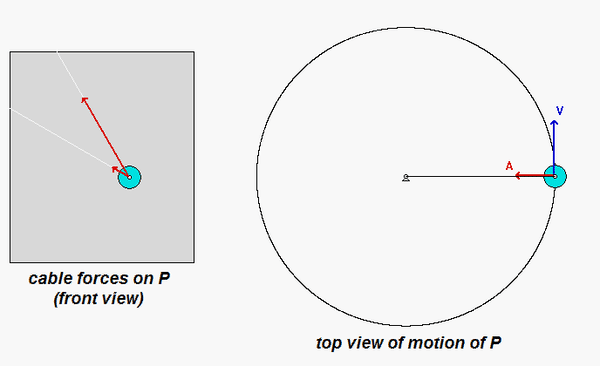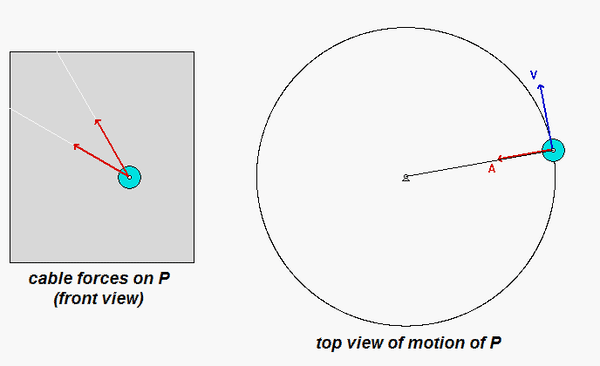# Influence of speed on cable tensionsTwo wires, OP and AP, connect particle P (having a mass of m) to fixed points O and A, respectively, where OA is a vertical line. The particle rotates about axis OA such that P has a constant speed of vP and with the two wires remaining taut as particle moves on a circular path of radius R

What do we know from the start about the influence of the speed and the weight of P on the tension in the two wires?

• If the speed of P is increased, P will want to swing outward. This will increase the tension in wire AP but decrease the tension in wire OP. For too large of a rotation rate, wire OP will go slack.
• If the weight of P is increased, P will want to swing downward. This will increase the tension in wire OP but decrease the tension in wire AP. If the weight of P is increased too much, wire AP will go slack.
• Summarizing above, if the speed of P is too large, wire OP will go slack. If the speed of P is too small, wire AP will go slack.

Consider the animations below from three simulations for which both wires remain taut: a slow rotation rate, an intermediate rotation rate and a fast rotation rate.

Slow rotation rateIntermediate rotation rateFast rotation rateObservations: These three animations confirm our original assessment that as the speed is increased, the tension in wire AP increases, and the tension in wire OP decreases.